### MEMO 2007 ekipno problem 7

Kvaliteta:
Avg: 2,3
Težina:
Avg: 6,0
A tetrahedron is called a MEMO-tetrahedron if all six sidelengths are different positive integers where one of them is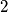$2$ and one of them is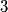$3$. Let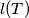$l(T)$ be the sum of the sidelengths of the tetrahedron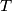$T$.
(a) Find all positive integers$n$ so that there exists a MEMO-Tetrahedron$T$ with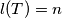$l(T)=n$.
(b) How many pairwise non-congruent MEMO-tetrahedrons$T$ satisfying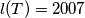$l(T)=2007$ exist? Two tetrahedrons are said to be non-congruent if one cannot be obtained from the other by a composition of reflections in planes, translations and rotations. (It is not neccessary to prove that the tetrahedrons are not degenerate, i.e. that they have a positive volume).
Izvor: Srednjoeuropska matematička olimpijada 2007, ekipno natjecanje, problem 7